# 前言

• 第一人称模式 -- 操作就类似行人或车在行进的效果，可以通过键盘鼠标控制前进后退。

• 自动巡检模式 -- 该模式下用户不需要任何操作，场景自动前进后退来巡查当前地铁站的场景。

• 鼠标操作模式 -- 左键旋转场景，右键平移场景。

# 界面简介及效果预览

### 地铁运行效果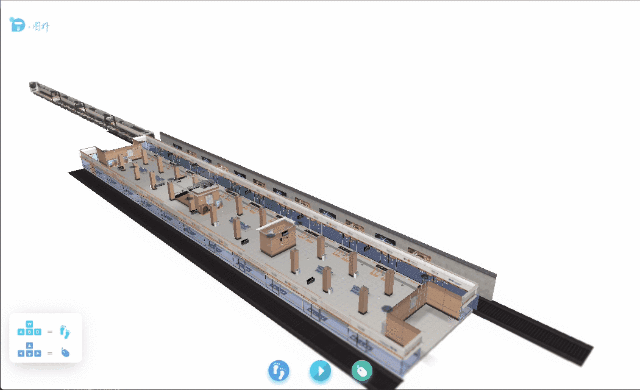### 漫游效果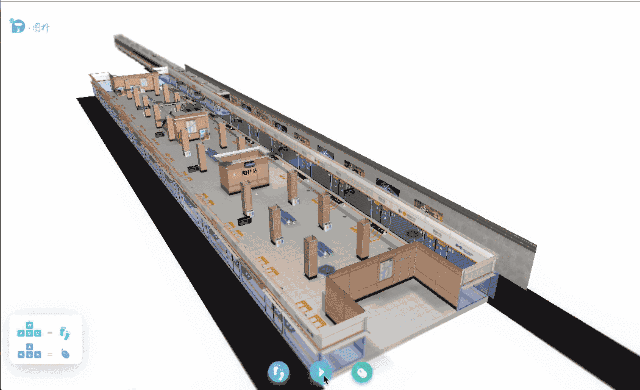### 监控设备交互效果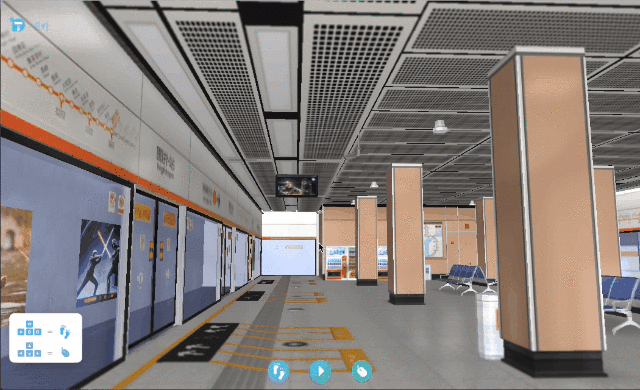# 场景搭建

``// 分别为 obj 文件地址，mtl 文件地址ht.Default.loadObj('obj/metro.obj', 'obj/metro.mtl', {    center: true,    // 模型是否居中，默认为 false，设置为 true 则会移动模型位置使其内容居中r3: [0, -Math.PI / 2, 0],    // 旋转变化参数，格式为 [rx, ry, rz]s3: [0.15, 0.15, 0.15],    // 大小变化参数，格式为 [sx, sy, sz]finishFunc: function(modelMap, array, rawS3) {        if (modelMap) {            ht.Default.setShape3dModel('metro', array); // 注册一个名字为 metro 的模型        }    }});``

``var node = new ht.Node();node.s({    'shape3d': 'metro'});``

# 动画代码分析

### 地铁动画代码的实现分析

``let metroTask = {    interval: 50,    // 每五十秒执行一次action: (data) = >{ // 即上文所提回调函数// 判断当时传进来的节点是否为地铁列车节点if (data === currentMetro) {            // 获取地铁此时的 X 轴位置以及行进的方向let currentX = data.getX(),            direction = data.a('direction');            // 根据当前的 X 轴位置获取当前的列车速度let speed = this.getSpeedByX(currentX);            // 根据当前的 X 轴位置获取当前的列车透明度let opacity = this.getOpacityByX(currentX);            // 判断此时 X 轴位置是否超过某个值 即地铁是在某个范围内移动if (Math.abs(currentX) <= 5000) {                // 设置当前的透明度                opacity !== 1 ? currentMetro.s({                    'shape3d.transparent': true,                    'shape3d.opacity': opacity                }) : currentMetro.s({                    'shape3d.transparent': false                });                // 设置当前的 X 轴位置                data.setX(currentX + direction * speed);                // 判断此时地铁的速度为 0，所以此时应该执行开门的动画if (speed === 0) this.doorAnimation(currentMetro, direction);            }            // 右方向地铁开到头，进行复位if (currentX > 5000 && direction === 1) {                currentMetro = leftMetro;                currentMetro.setX(5000);            }            // 左方向地铁开到头，进行复位if (currentX < -5000 && direction === -1) {                currentMetro = rightMetro;                currentMetro.setX( - 5000);            }        }    }};dm3d.addScheduleTask(metroTask);``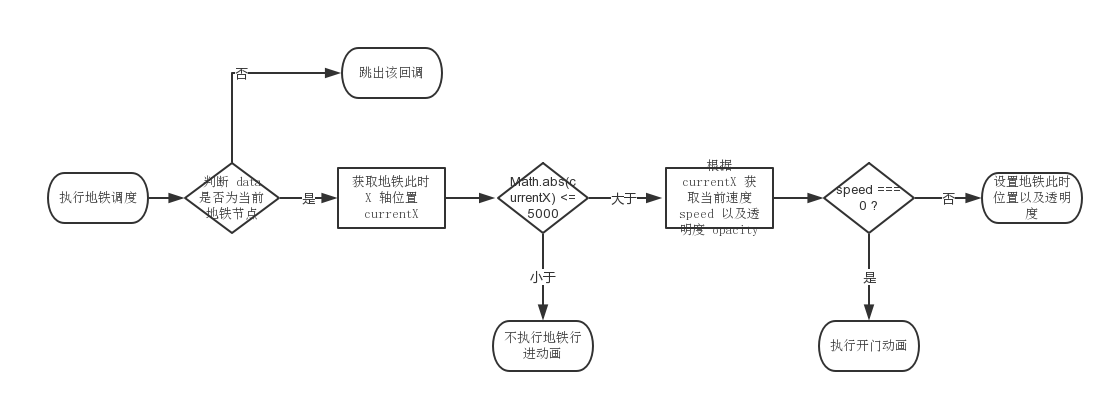``currentMetro.setX(direction * 10); // 设置出站列车的位置``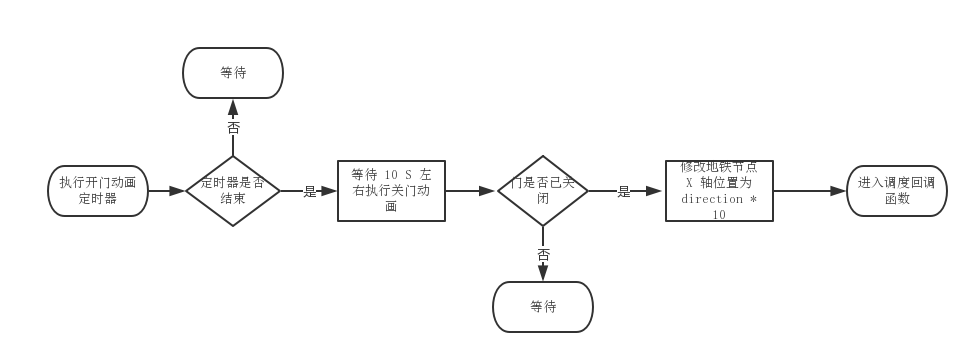### 自动巡检代码的实现分析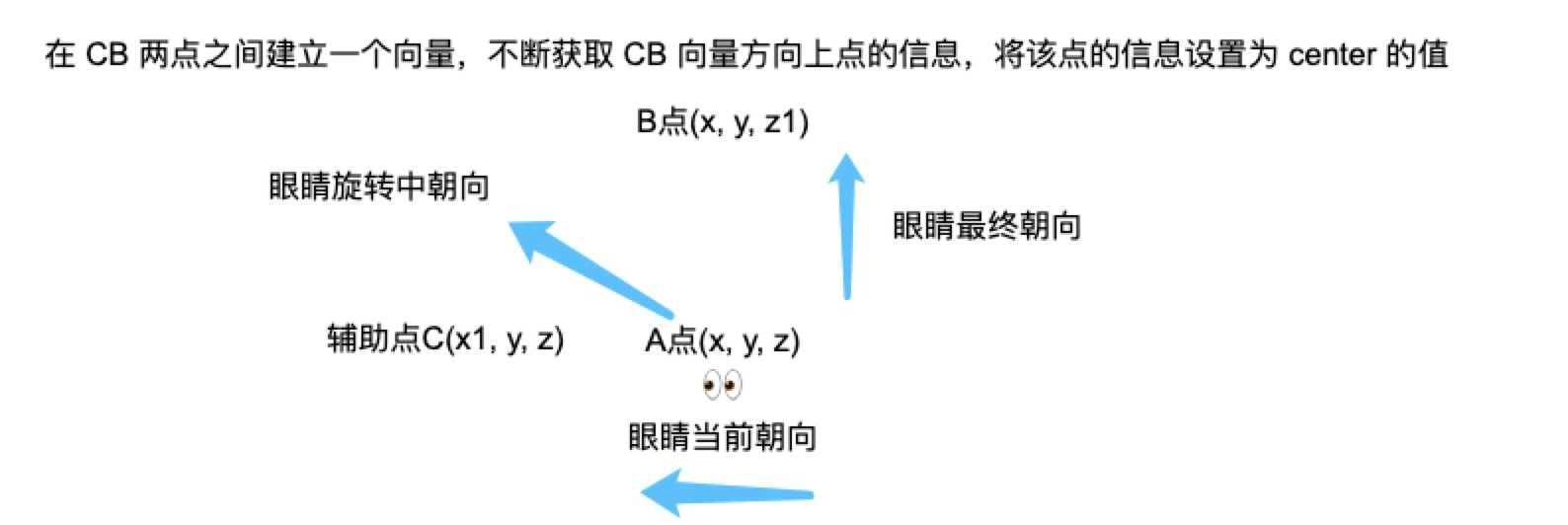``rotateStep() {    // 即上图辅助点 Clet fromCenter = this.fromCenter;    // 即上图 B 点let toCenter = this.toCenter;    // 每帧转一度let rotateValue = this.rotateFrame || Math.PI / 180;    // 辅助点 C 与 B 点之间建立一个方向向量let centerVector = new ht.Math.Vector2(toCenter.x - fromCenter.x, toCenter.y - fromCenter.y);    let centerVectorLength = centerVector.length();    // 此时旋转百分比let rotatePercent = rotateValue * this.stepNum / this.curRotateVal;    if (rotatePercent >= 1) {        rotatePercent = 1;        this.stepNum = -2;    }    let newLength = rotatePercent * centerVectorLength;    centerVector.setLength(newLength);    let newCenterVector = centerVector.add(fromCenter);    // 获取旋转过程中 center 的点信息let newCenterPosition = [newCenterVector.x, this.personHeight, newCenterVector.y];    // 设置当前 center 的大小this.g3d.setCenter(newCenterPosition);}``

### 电梯动画代码的实现分析

``// vs 指的是构成电梯模型所有的三角面顶点坐标数组// 由于场景中电梯的运行方向为往对角线右上方运动，所以只需要修改 x 轴以及 y 轴坐标值// xStep yStep 为每次电梯运动的距离setInterval(() = >{    // i+3 是因为 vs 数组的顺序为 x, y, z 轴 所以每次 i 偏移三个单位大小for (let i = 0, l = vs.length; i < l; i = i + 3) {        // 该顶点坐标下一个 x 轴坐标的值let nextX = vs[i] - xStep;        // 该顶点坐标下一个 y 轴坐标的值let nextY = vs[i + 1] + yStep;        vs[i] = nextX < -0.5 ? 0.5 - (Math.abs(nextX) - 0.5) : nextX;        vs[i + 1] = nextY > 0.5 ? -0.5 + (Math.abs(nextY) - 0.5) : nextY;    }},200);``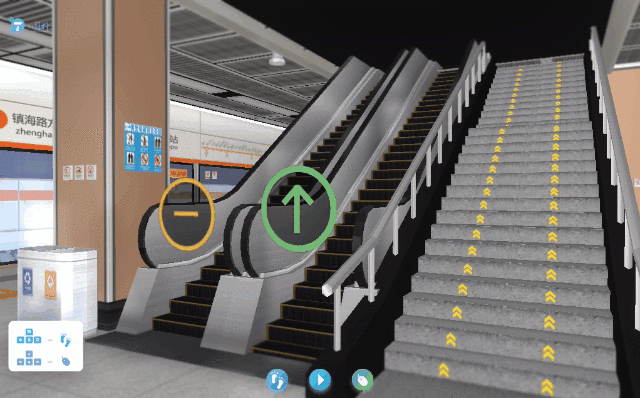# 监控功能展示及介绍

### 视频监控### 烟雾报警监控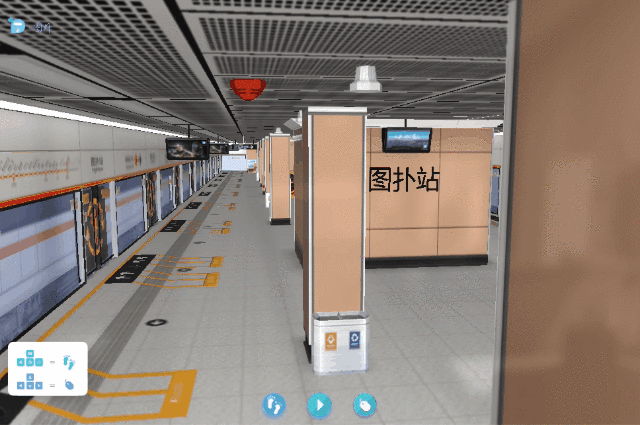### 电视列车到站时间监控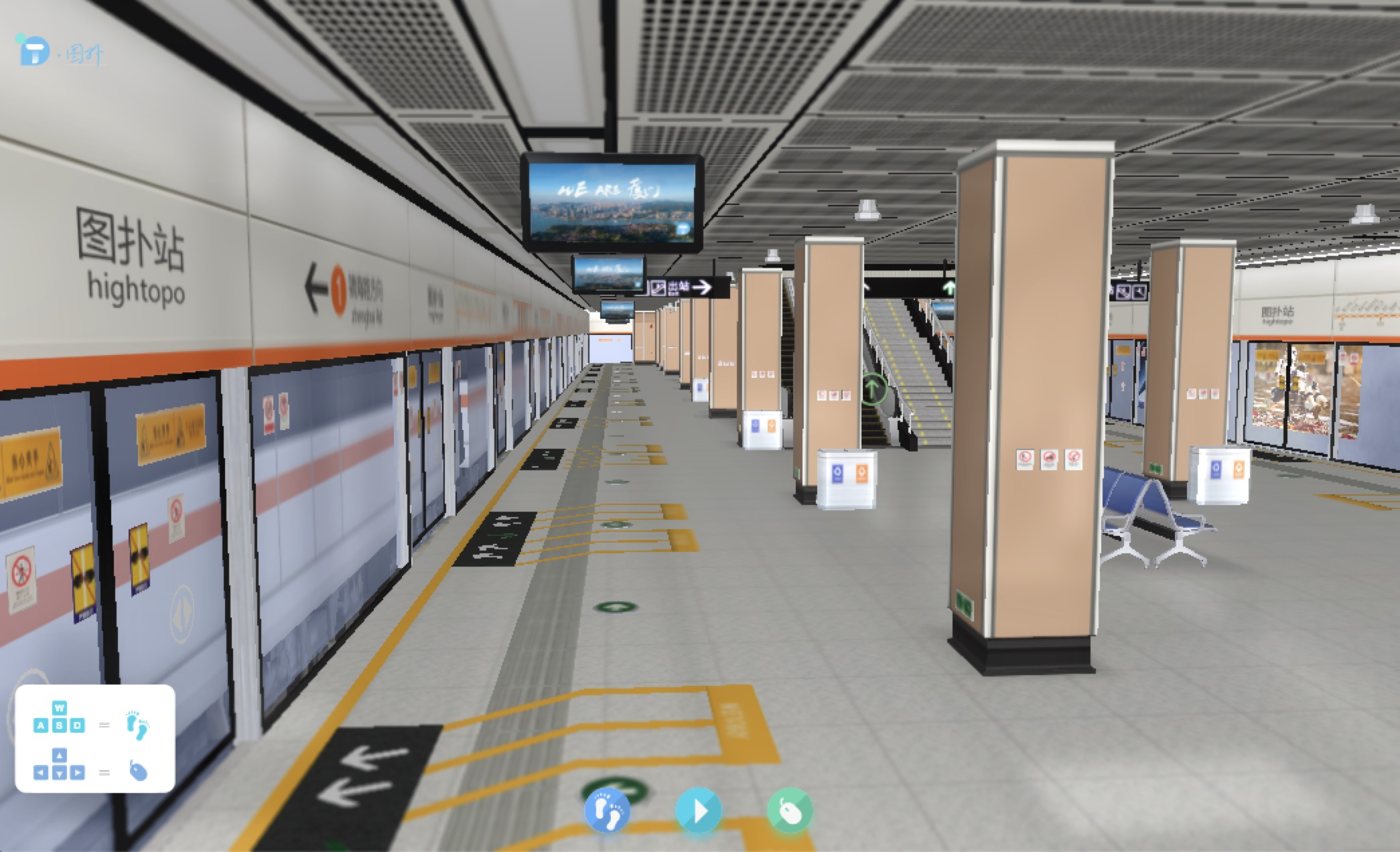### 场景监控交互

3D 场景中交互是比较简单的，主要是点击摄像头展示 2D 监控面板，在 2D 界面中主要是切换三种交互模式，三种交互模式为互斥的关系，以下是 3D 交互注册事件代码:

``g3d.mi((e) = >{    let {        g2d,        dm2d    } = this;    // 为点击类型if (e.kind === 'clickData') {        // data 为当前点击的图元let data = e.data;        // 当前图元的 shape3d 类型let shape3d = data.s('shape3d');        // 判断当前 shape3d 类型是否为摄像头if (shape3d && shape3d.indexOf('摄像头') > 0) {            let cameraPanel = dm2d.getDataByTag('cameraPanel');            // toggle 切换摄像头 2d 面板            g2d.isVisible(cameraPanel) ? cameraPanel.s('2d.visible', false) : cameraPanel.s('2d.visible', true);        }    }    // 为点击 3d 场景背景类型if (e.kind === 'clickBackground') {        let cameraPanel = dm2d.getDataByTag('cameraPanel');        // 隐藏摄像头 2d 面板        g2d.isVisible(cameraPanel) && cameraPanel.s('2d.visible', false);    }});``

# 总结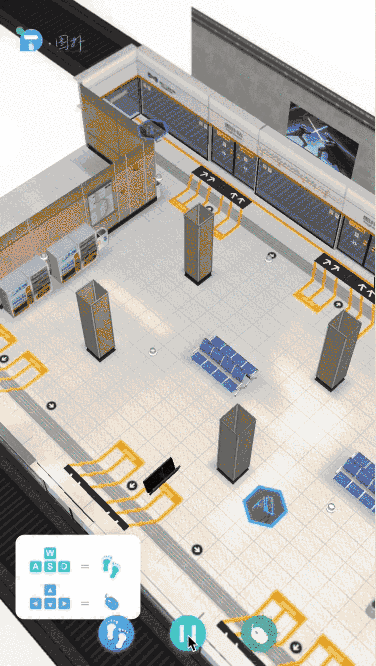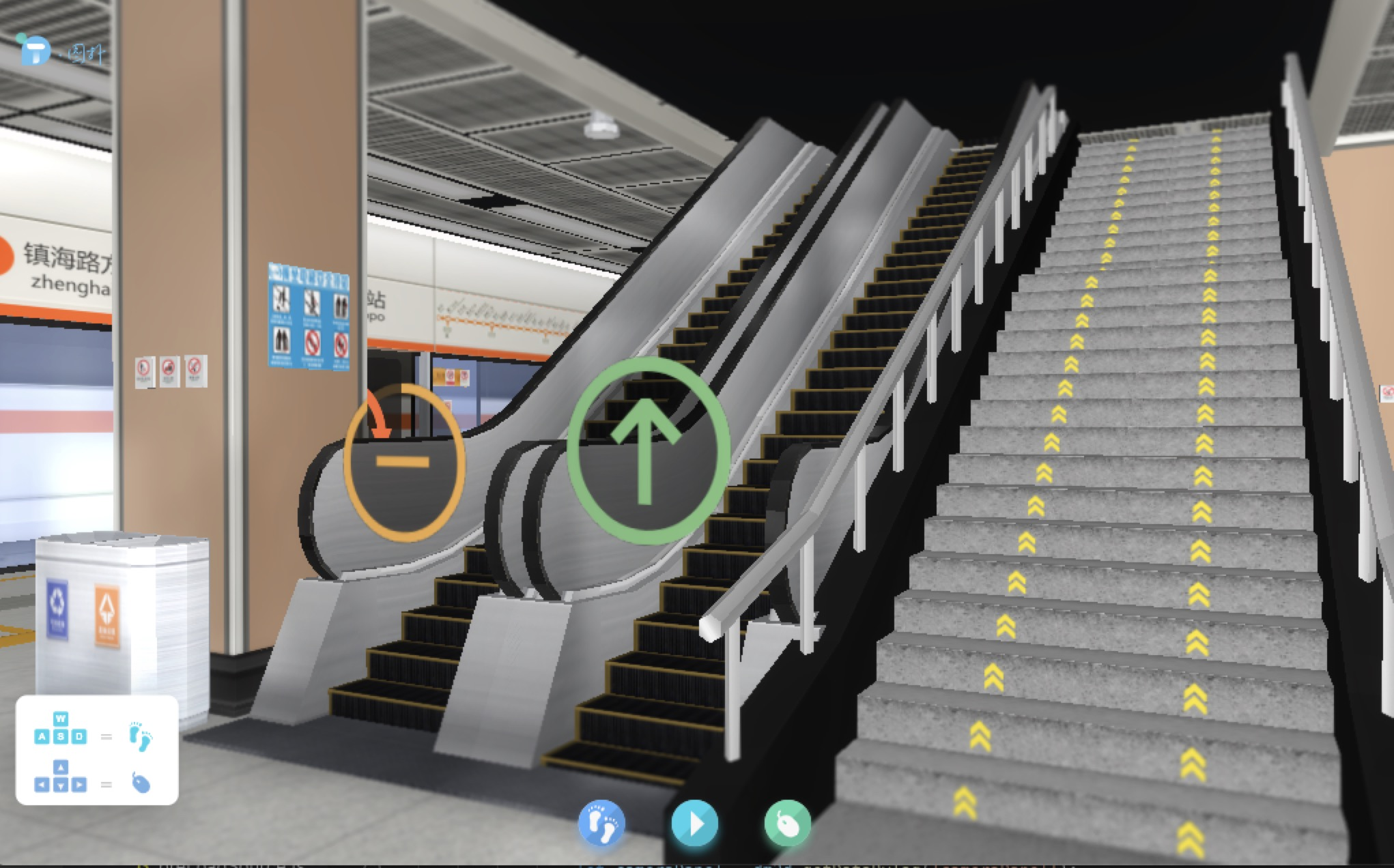I 专注WEBGL图形处理技术的研究与应用